The effectiveness of a pad and fan system will not only depend on the design, but also on the wet bulb temperature outside the greenhouse. It is not the relative humidity, as many grower think that determine the effectiveness of the pad and fan system. The wet bulb temperature will determine to what temperature the air can be cooled by the evaporation of water. The wet bulb temperature can be obtained from The South African Weather Bureau.

A pad and fan system consists of:

• One or more extraction fans depending on the size of the greenhouse
• A large pad which varies in size depending of various factors
• A water supply system with a sump tank in which water can be stored

The effectiveness of a pad and fan system will also depend on how air tight the greenhouse is. This is important because the more air is sucked through the wet pads, the higher the efficiency of the pad and fan system. Thus maintenance of the greenhouse cladding is extremely important when installing a pad and fan system.

The calculations found below are based on those of Ausberger, Bohanon, et al. (1975). Although the original calculations were in feet, the formulas have been converted to SI units. The following data is required in calculating the pad and fan requirements:

• Elevation of the greenhouse operation
• The light intensity of the area
• The temperature differential required
• Length and width of the greenhouse

The elevation of the greenhouse is important since the heat removal capacity of air depends on its weight and not in its volume. Air is less dens at higher altitudes, so the elevation must be included in calculations. A method of calculating the elevation factor (Felev) is provided in the equation below. The constant “e” has a value of 2.7182818…

Elevation in meter (SI):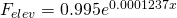Elevation in feet (ft):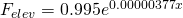The light intensity will influence the amount of energy reaching the greenhouse thus will determine how quickly and to what temperature the greenhouse will be heated. The factor for light intensity (Flight) can be determined in various ways:

Using foot candles: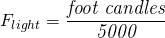If foot candle values are not available, other units can be used such as “lux” in which the constant (5000) must be replaced with 53819. Another less known unit is “phot”, in which case the constant (5000) must be replaced with 5.382.

Another crude method of calculating the light intensity factor is using the average heat units between October and March (HUOM)for the specific area in which the greenhouse is located, and dividing it with the average heat units for the southern hemisphere for the same period. Thus the formula is as follows: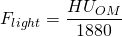The temperature difference that needs to be achieved between the pad and fan inside the greenhouse needs to be determined beforehand. This depends on the environment and crop requirements and type. If the difference is less than 4 °C, Ftemp equals 1.00, otherwise use the following formula: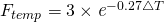The value of e = 2.7182818 which is a universal constant used in biological mathematics. All these factors determine the factor that needs to be used to adjust for the greenhouse design (Fhouse)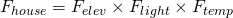The next step is to determine the pad to fan distance factor (Fvel). Use the following formula: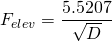The velocity factor (Fvel) and greenhouse design factor (Fhouse) is used when the pad to fan distance is equal or less the 30 m. For greenhouses longer than 30 m, only the Fhouse factor is used in further calculations. The pad to fan distance should never exceed 60 m. If the greenhouse is longer than 60 m, the grower should try and place the pads along the length of the greenhouse and the fans on the opposite side. This will require more fans however, but it will increase the efficiency of the system. The fans should not be placed further than 8 m from each other.

The total air flow (m3.min-1) required for a greenhouse less than 30 m long (AF<30)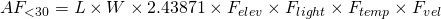Calculation of air flow for a greenhouse longer than 30 m (AF):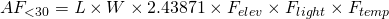The following example will illustrate the use of above formulas in order to calculate the air flow of a single span greenhouse with the following dimensions and data:

• Width: 8 m
• Length: 30 m
• Elevation: 1,500 m
• Average heat units: 2,450
• Temperature difference required: 3°C
• Pad to fan distance: 30 m

The following calculations are made using and above equations: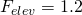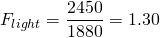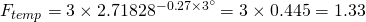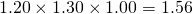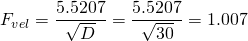Since the structure is less than 30 m, AF&lt30 will be used in order to calculate the air flow requirements. The Ftemp value is calculated as 1.33, but since the required difference is less than 4 °C, Ftemp is equal to 1.00.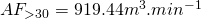The next step is to determine the size of the pad that will be used in the greenhouse. There are basically two methods, each providing a slightly different result. The first method uses the distance between pad and fan. According to the European and American standards the height of the pad increases 1 unit with each 20-28 units of distance between pad and fan. So for a 30 m tunnel the height of the pad must be 1.07 m (using the 1:28 ratio). However, with an arid climate it is recommended that the ratio should be altered from 1:28 to at least 1:20. This means that for a tunnel of 30 m the pad height should be 1.6 m. The total area of the pad would be, using above example, 8 m x 1.6 m = 12.8 m².

The second method is based on the required air flow of the system as calculated above. It takes into consideration the temperature difference from pad to fan is slightly more accurate. The following calculations are required in order to calculate the required pad size. Calculating the area of the evaporative pad (PA) according to the required air flow of the greenhouse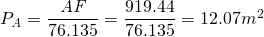The total required evaporative pad area is provided by PA, the height of the pad will depend on the width of the pad (PW) and is usually as wide as the greenhouse (W) itself. So W = PW. Calculating the height of the evaporative pad (PH) according to the width of the pad (PW):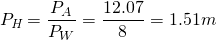So the height of the evaporative pad should be 1.51 m.

The next step is to calculate:

• the required flow rate for the evaporative pad
• The sump size to supply and maintain enough water for the evaporative pad and
• The estimated water consumption

It is important to determine the required flow rate for the evaporative pad since that will provide a guideline as to which pump should be purchased. Calculating the required flow rate (PF) of the water feeding the evaporative pad.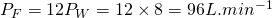The next step is to calculate the sump size. Calculating the required sump size (PS) for the evaporative pad.The next step is to calculate the estimated water loss per minute from the evaporative pad. This will provide the grower a good indication of how much water he should budget for. Calculating the estimated water consumption (PW) of the evaporative pad.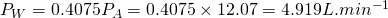It is thus estimated that a maximum of 5 L.min-1 of water is required to maintain the water level in the sump. Remember this is a maximum value and if the supply line can manage 5 L.min-1, the sump will not run dry. The temperature of the water will have an insignificant effect on the cooling ability of the evaporative pad. This is because the cooling ability depends on the wet bulb temperature and not the dry bulb temperature, water temperature or relative humidity.